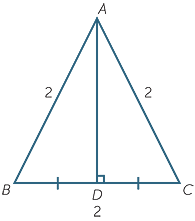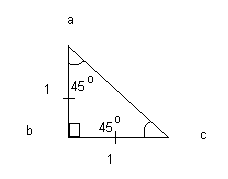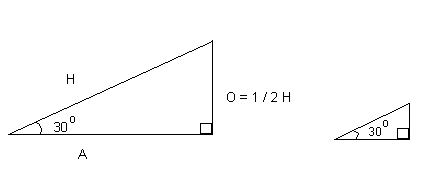# Application of Surds to Trigonometric Ratios

Welcome to class!

In today’s class, we will be talking about the application of surds to trigonometric ratios. Enjoy the class!

### Application of surds to trigonometric ratiosApart from solving quadratics, surds also arise in trigonometry.

The angles 30°, 45°, 60° have the following trigonometric ratios.

Triangle ABC is equilateral. AD is the line interval from A to the midpoint of BD. Triangles ABD and ACD are congruent (SSS).

Hence,

 sin 30° = cos 60° = 1/2 , sin 60° = cos 30° = $\surd 3/2$  , tan 30° = $1/\surd 3$   and tan 60° = $\surd 3$  .

#### The trigonometric functions of y = cos θ and y = cos θ.

 θ° 0 30 60 90 120 150 180 210 240 270 300 330 360 sin θ 0 0.5 0.87 1 0.87 0.5 0 −0.5 −0.87 −1 −0.87 −0.5 0

More points can be used to show that the shape of the graph is as shown below.Electrical engineers and physicists call this a sine wave.The basic formulae here all reply on the SINE graph as follows:

• The sine of an angle is defined by the vertical height of a point as it rotates around a unit circle (that is, its radius is 1) measured from a horizontal line through the centre of the circle. So it cannot be bigger than 1 or less than -1.
• the cosine of an angle is defined by the horizontal distance of a point as it rotates around the unit circle measured from a vertical line through the centre of the circle. It too must be in the range -1 to 1.

#### The surd form of trigonometric ratios:The equilateral triangle above has sides each 2 units long and all angles at 60o. It has been halved into 2 right – angled triangles of base 1 unit long. Using Pythagoras:

(ab)2 = (ac)2 + (bc)2
=> (2)2 = (ac)2 + (1)2
=> (ac)2 = 4 – 1 = 3
and (ac) = root 3.

Using the definitions of trigonometric ratios, summarised in SOHCAHTOA:

sin 30o = bc / ab = 1 / 2
sin 60o = ac / ab = root 3 / 2
cos 30o = ac / ab = root 3 / 2
cos 60o = bc / ab = 1 /2
tan 30o = bc / ac = 1 / root 3
tan 60 = ac / bc = root 3 / 1 = root 3The isosceles above has equal sides of 1 unit each and the 2 complementary angles each of 45o. Using Pythagoras’ Theorem to find side (ac):

(ac)2 = (ab)2 + (bc)2
(ac)2 = (1)2 + (1)2 = 2
ac = root 2

 Trigonometrical ratio: Surd form: Approximation sin 30o 1 / 2 0.5 sin 45o 1 / root 2 0.7071 sin 60o root 3 / 2 0.866 cos 30o root 3 / 2 0.866 cos 45 1 / root 2 0.7071 cos 60o 1 / 2 0.5 tan 30o 1 / root 3 0.5774 tan 45o 1 tan 60o root 3 1.7321
##### For any pair of complementary angles these 2 rules apply:

sin x = cos (90 – x)
and cos x = sin (90 – x)

 sin 30o = cos 60o sin 60o = cos 30o sin 45o = cos 45o sin 20o = cos 70o cos 41o = sin 49o cos 72o = sin 18o sin 36o = cos 54oWhen the angle is 30o on a right-angled triangle, then the side opposite the 30o angle is half the hypotenuse. This is also true for similar triangles. Sides H, A and O can be any value and O = 1 / 2 H. Therefore, sin 30o = 0.5.

In our next class, we will be talking about Matrices and Determinant. We hope you enjoyed the class.

Should you have any further question, feel free to ask in the comment section below and trust us to respond as soon as possible.

How Can We Make ClassNotesNG Better - CLICK to Tell Us💃

1.